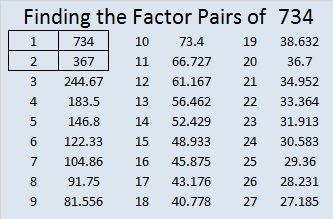# There Are 10 Ways 734 is the Sum of 3 Squares

• 734 is a composite number.
• Prime factorization: 734 = 2 x 367
• The exponents in the prime factorization are 1 and 1. Adding one to each and multiplying we get (1 + 1)(1 + 1) = 2 x 2 = 4. Therefore 734 has exactly 4 factors.
• Factors of 734: 1, 2, 367, 734
• Factor pairs: 734 = 1 x 734 or 2 x 367
• 734 has no square factors that allow its square root to be simplified. √734 ≈ 27.092434.Here is a factoring puzzle for you to try. If you need some hints, scroll down the page.Print the puzzles or type the solution on this excel file: 10 Factors 2016-01-04

———————————————————

Here’s a little more about the number 734:

182 + 183 + 184 + 185 = 734; that means 734 is the sum of 4 consecutive numbers.

OEIS.org informs us that 734 is the smallest number that can be expressed as the sum of 3 square numbers 10 different ways. I couldn’t resist the challenge of finding what those 10 ways are:

1. 27² + 2² + 1² = 734
2. 26² + 7² + 3² = 734
3. 25² + 10² + 3² = 734
4. 23² + 14² + 3² = 734
5. 23² + 13² + 6² = 734
6. 22² + 15² + 5² = 734
7. 22² + 13² + 9² = 734
8. 21² + 17² + 2² = 734
9. 19² + 18² + 7² = 734
10. 18² + 17² + 11² = 734

734 is palindrome 23132 in BASE 4; note that 2(4^4) + 3(4^3) + 1(4^2) + 3(4^1) + 2(4^0) = 734

———————————————————

A Logical Approach to solve a FIND THE FACTORS puzzle: Find the column or row with two clues and find their common factor. (None of the factors are greater than 10.)  Write the corresponding factors in the factor column (1st column) and factor row (top row).  Because this is a level three puzzle, you have now written a factor at the top of the factor column. Continue to work from the top of the factor column to the bottom, finding factors and filling in the factor column and the factor row one cell at a time as you go.